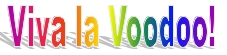Faqs | Help | @ | Contact |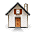## A Short Second Opinion on Info-Gap Decision Theory

The objective of this short note is to provide a general, non-technical summary of what is amiss with Info-Gap decision theory. An extended discussion outlining in great detail the true nature of Info-Gap Decision Theory and its profound failings can be found at:

info-gap.moshe-online.com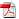A PDF version of this file is available.The report is not long. But ... if you are pressed for time, you may want to read the conclusions first.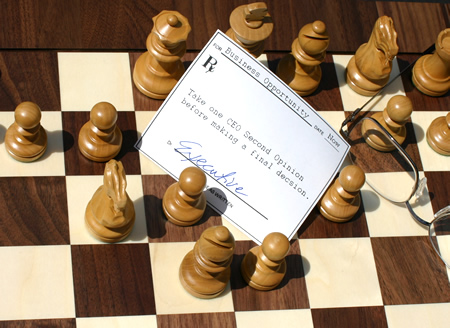This discussion is intended to give analysts and decision makers who have fallen for Info-Gap decision theory’s rhetoric a "second opinion". It’s objective is to make it clear that Info-gap's rhetoric gives a false impression that it provides a sound scientific paradigm for robust decision-making in the face of severe uncertainty.

The main objective of the report is to set the record straight on a number of misconceptions that are propagated in the Info-Gap literature.

1. Info-Gap decision theory misrepresents the facts about its role and place in decision theory. In particular, the contention that it is a distinct, novel, revolutionary and radically different methodology for the treatment of severe uncertainty is grossly in error.

The fact of the matter is that Info-Gap's robustness model is a simple instance of none other than the most celebrated model in Classical Decision Theory for the treatment of severe uncertainty, namely: Wald's Maximin/Minimax model (circa 1940). Likewise, Info-Gap's opportuneness model is a simple instance of the well known (super-optimistic) Minimin model (circa 1950).

The repeated futile attempts in the Info-Gap literature to deal with this embarrassing fact only expose Info-Gap decision theory to harsher criticism. Indeed, these attempts exhibit even deeper conceptual and technical errors.

2. Ironically, the trait that does set Info-Gap apart from other methods in this field is the fundamentally flawed manner in which its Maximin and Minimin models are deployed in the treatment of severe uncertainty. Indeed, this makes a mockery of Info-gap's declared objective: the treatment of severe uncertainty.

Info-Gap's basic contention is that its robustness model is designed specifically to seek robust decisions for problems that are subject to the severest uncertainty imaginable: "true Knightian Uncertainty". But the fact of the matter is that this model is in principle unable to deliver on this declared aim because of its peculiar mode of operation, namely the local nature of the analysis that it prescribes.

That is, Info-Gap's Maximin/Minimin models use a single point estimate of the parameter of interest as the focal point of the robustness and opportuneness analyses. At the same time, the fundamental working assumption of Info-Gap decision theory is that under conditions of severe uncertainty the estimate is a wild guess of the true value, and is likely to be substantially wrong. So what do these analyses amount to? The picture is this:

 No Man's LandNo Man's Land

where

• The tiny white dot represents the wild guess of the true value of the parameter of interest.

• The black area around the wild guess represents the region of uncertainty affecting Info-Gap's robustness/opportuneness analysis.

• The light gray area represents Info-Gap's No Man's Land: that part of the uncertainty space that has no impact whatsoever on Info-Gap's robustness/opportuneness analysis.

So, how worthwhile/meaningfull/valid can results yielded by an analysis in the immediate neighborhood of the estimate be?

Indeed, not only is there no basis whatsoever to expect these results to be reliable, the analysis gives the decision-maker a thoroughly distorted picture of how the severity of the uncertainty is tackled by Info-Gap decision theory.

In a nutshell, the vaunted Info-Gap approach to uncertainty prescribes an analysis that effectively violates the universally accepted Garbage in - Garbage out Maxim. For, what Info-Gap's basic claim comes down to is that it is capable of accomplishing the following feat:

 wild guess ----------> Info-Gap Decision Theory ----------> robust decision

This renders Info-gap decision theory a voodoo decision theory par excellence.

3. This glaring flaw is further exacerbated in cases where the region of uncertainty under consideration is unbounded. Note then that an unbounded uncertainty space is — according to the Info-Gap literature — its commonly encountered case. Furthermore, it is the factor distinguishing Info-Gap decision theory from other non-probabilistic approaches to severe uncertainty.

The point is then that the proposition to rely on a local analysis of the neighborhood of a wild guess of the true value of the parameter of interest in such an environment — where the uncertainty space is unbounded — is absurd. Because, the picture is this:

 -∞ <---- No Man's LandNo Man's Land ----> ∞

This means that Info-Gap decision theory in effect argues that a local analysis in a tiny area around a wild guess is capable of yielding decisions that are robust relative to an unbounded region of uncertainty!

The absurd is so obvious that all that is left to say is that this is not science. This is voodoo science par excellence.

The main point is that Info-Gap decision theory’s prescription for the management of the severe uncertainty is to . . . simply ignore it

The following picture illustrates this point.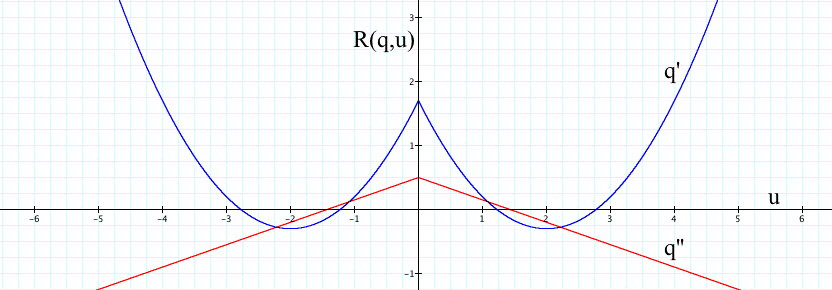It shows the rewards R(q,u) generated by two decisions, q' and q'', as a function of some parameter u. The estimate of the true value of u is û = 0, the uncertainty space is U=(-∞,∞) and the performance requirement is R(q,u) ≥ 0.

According to Info-Gap's robustness model, q'' is more robust than q' with respect to R(q,u) ≥ 0 because the closest u to û that violates the constraint R(q',u) ≥ 0 is at a distance α'=1.08 from û, whereas the closest u to û that violates the constraint R(q'',u) ≥ 0 is at a distance α''=1.429 from û. Hence, q'' is more robust than q'.

Note, however, that

• R(q',u) > R(q'',u) almost everywhere on U, except for the two small intervals of [1.08,2.22] and [-2.22,-1.08].

• q'' violates the robustness condition R(q'',u) ≥ 0 almost everywhere on U, except for the small interval [-1.429,1.429].

• q' satisfices the robustness condition R(q',u) ≥ 0 almost everywhere on U, except for the small intervals [1.23,2.78] and [-2.78,-1.23].

This example also illustrates why Info-Gap's robustness analysis cannot handle ordinary, plain, white Swans, let alone genuine (Australian) Black Swans.

4. Info-Gap decision theory contends that methodologies seeking to optimize reward do not yield robust decisions. The claim is that strategies based on reward optimization yield decisions with zero robustness to uncertainty.

This alleged serious shortcoming of optimization models — according to Info-Gap decision theory — gives Info-Gap its point and merit. For, in contrast, Info-Gap seeks decisions that are robust-satisficing, rather than reward-optimizing.

But as anyone even mildly conversant with Optimization Theory would no doubt know, this is a grossly erroneous thesis.

To begin with, the robustness — as prescribed by Info-Gap — of decisions that optimize reward is typically not zero. For example, as shown above, the robustness of the reward maximizing decision q' at û is not zero. In fact, there are many cases where reward optimizing decisions are the very decisions that Info-Gap itself deems to be the most robust. Secondly, if one's goal is to obtain robust decisions, then constrained optimization provides precisely the means for this purpose: mathematical models that enable the incorporation of robustness in the formulation of the optimization models, let alone techniques to solve optimization problems defined by such models. Indeed, this is precisely what is being done, for some 40 years now, in the thriving field of Robust Optimization.

So, not only is it the case that Info-Gap decision theory gives a totally distorted view of how robustness is handled by optimization theory, it consistently, persistently, and deliberately refrains from referring to the very rich literature on Robust Optimization.

But why?

5. Pursuant to its erroneous position on optimization theory's purported inability to yield robust decisions, Info-Gap decision theory then proceeds to argue that Info-Gap's so called "robust satisficing strategy" is superior to what it calls "direct optimization" strategies.

More generally, the argument is that "satisficing" has an inherent advantage on "optimizing".

This muddled thesis not only fails to show the advantage of Info-Gap's so-called "robust-satisficing strategy", but it effectively exposes profound misconceptions about the whole satisficing vs. optimizing debate. For one thing, it betrays a lack of knowledge/understanding of the fact that any satisficing problem can be easily formulated as an equivalent optimization problem. The implication is then that the "satisficing vs optimizing" issue does not boil down to whether satisficing is superior to optimizing. This is a non-issue.

Rather, the issue in this debate is: what should be optimized and what should be satisficed?

But, for all its rhetoric, Info-Gap decision theory does not shed any light whatsoever on this valid question.

Conclusions

• Contrary to persistent claims by proponents of Info-Gap decision theory, Info-Gap's Robustness model and Opportuneness model are neither new nor radically different from models used in classical decision theory and robust optimization:

• Info-Gap's robustness model is a simple Maximin model (circa 1940).

• Info-Gap's opportuneness model is a simple Minimin model (circa 1950).

• Info-Gap's local implementation of these models around a wild guess is thoroughly unsuitable for the treatment of severe uncertainty as it violates the Garbage In - Garbage Out Maxim.

• Info-Gap's purported ability to deal with an unbounded uncertainty space is not due to some secret weapon. The irony is that this claims comes to naught because of the theory's inherently local approach to uncertainty. That is, by focusing the analysis on a (given) point estimate of the parameter of interest and its immediate neighborhood, Info-Gap's robustness analysis effectively ignores the vast uncertainty space (the severity of the uncertainty) that it postulates.

• Info-Gap's pronouncements regarding the "satisficing vs optimizing" debate are erroneous and counter-productive.

• Info-Gap's deliberate disregard of the state of the art in Robust Optimization is inexplicable and inexcusable.

In short, peeling off the layers of rhetoric from the Info-Gap enterprise reveals a theory that employs a Maximin robustness model and a Minimin opportuneness model in the neighborhood of a wild guess of the true value of the parameter of interest. This theory is therefore unsuitable for decision-making under severe uncertainty. Indeed, it is a classic example of a voodoo decision theory.

A more detailed technical critique of Info-Gap decision theory is available online at:

and in the references listed therein, including the entry Info-Gap Decision Theory in WIKIPEDIA.

Postscript:

Now, with the publication of a fourth book on Info-Gap decision theory (see Info-Gap Economics), it is high time that the Father of Info-Gap decision theory explain clearly a number of facts.

A good starting point would be a clear answer to the following simple question:

What exactly is the difference between Info-Gap's measure of robustness and the well-established Stability Radius that, for more than a quarter of a century, has been used extensively in control theory, as a measure of local robustness in the vicinity of a nominal value of the parameter of interest?!

In brief:

First, the math-free picture.

Consider a system that can be in either one of two states: a stable state or an unstable state, depending on the value of some parameter p. We also say that p is stable if the state associated with it is stable and that p is unstable if the state associated with it is unstable. Let P denote the set of all possible values of p, and let the "stable/unstable" partition of P be:

• S = set of stable values of p. We call it the region of stability of P.

• I = set of unstable value of p. We call it the region of instability of P.

Now, assume that our objective is to determine the stability of the system with respect to small perturbations in a given nominal value of p, call it p'. In this case, the question that we would ask ourselves would be as follows:

How much can we deviate from the nominal point p' (under the worst-case scenario) without leaving the region of stability S?

The "worst-case scenario" clause determines the "direction" of the perturbations in the value of p': we move away from p' in the worst direction. Note that the worst direction depends on the distance from p'. The following picture illustrates the simple concept behind this fundamental question.Consider the largest circle centered at p' in this picture. Since some points in the circle are unstable, and since under the worst-case scenario the deviation proceeds from p' to points on the boundary of the circle, it follows that, at some point, the deviation will exit the region of stability. This means then that the largest "safe" deviation from p' under the worst-case scenario is equal to the radius of the circle centered at p' that is nearest to the boundary separating the region of stability and the region of instability. And this is equivalent to saying that, under the worst-case scenario, any circle that is contained in the region of stability S is "safe".

So generalizing this idea from "circles" to high-dimensional "balls", we obtain:

The radius of stability of the system represented by (P,S,I) with respect to the nominal value p' is the radius of the largest "ball" centered at p' that is contained in the stability region S.

The following picture illustrates the clear equivalence of "Info-Gap robustness" and "stability radius":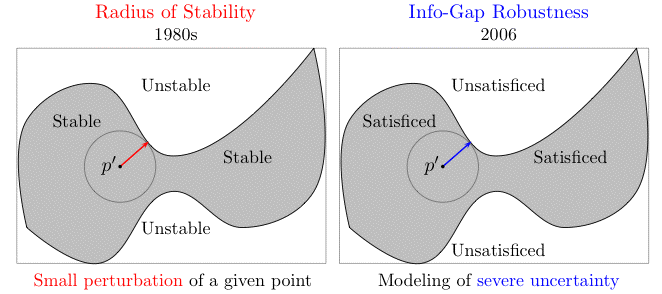In short, for all the spin and rhetoric, hailing Info-Gap's local measure of robustness as new and radically different, the fact of the matter is that this measure is none other than the "old warhorse" known universally as stability radius.

And as pointed out above, what is so objectionable about this state-of-affairs is not only that Info-Gap scholars fail to see (or ignore) this equivalence, but that those who should know better, continue to promote this theory from the pages of professional journals. See my discussion on Info-Gap Economics.

Math corner.

There are many ways to formally define the stability radius of a system. For our purposes here it is convenient to do it as follows:

 ρ(p')  := max {ρ≥0: p∈S, ∀p∈B(ρ,p')}

In words: the radius of stability is the largest value of ρ such that the ball B(ρ,p') centered at p' is contained in the region of stability S.

Now, condier the specific case where the region of stability S is defined by a performance constraint as follows:

 S  := {p∈P: r(d,p) ≤ r*}

where d denotes the system under consideration and r* is a given critical performance level.

Then in this case the stability radius of system q is as follow:

 ρ(d,p')  := max {ρ≥0: r(d,p) ≤ r*, ∀p∈B(ρ,p')}

In short:

Info-Gap's measure of Robustness   Stability Radius
 α(q,û)  := max {α≥0: r(d,u) ≤ r*, ∀u∈U(α,û)}
 ρ(d,p')  := max {ρ≥0: r(d,p) ≤ r*, ∀p∈B(ρ,p')}

The conclusion is therefore that Info-Gap's measure of robustness is a re-invention of the good old "stability radius".

So Ben-Haim and his followers should address the following simple questions:

• In what sense is Info-Gap decision theory a new theory that is radically different from all current theories on decisions under uncertainty?

• Given that its measure of robustness is by definition local in nature in that it is designed to measure small perturbations in a given nominal value of the parameter of interest, in what sense does Info-Gap decision theory "deal" with severe uncertainty, especially unbounded regions of uncertainty?

Remark:

In mathematics and control theory it is often more convenient to use the following alternative definition for the stability radius:

The radius of stability of the system represented by (P,S,I) with respect to the nominal value p' is the radius of the smallest "ball" centered at p' that contains an unstable point. That is, it is the distance from p' to the nearest unstable point in P.

In this case,

 ρ(p')  := inf {ρ≥0: ∃ p∈B(ρ,p') such that p∉S}

Note that the use of "inf" rather than "min" is due to the fact that a minimum value for ρ may not exist (eg. if I is an open set).

Disclaimer: This page, its contents and style, are the responsibility of the author (Moshe Sniedovich) and do not represent the views, policies or opinions of the organizations he is associated/affiliated with.